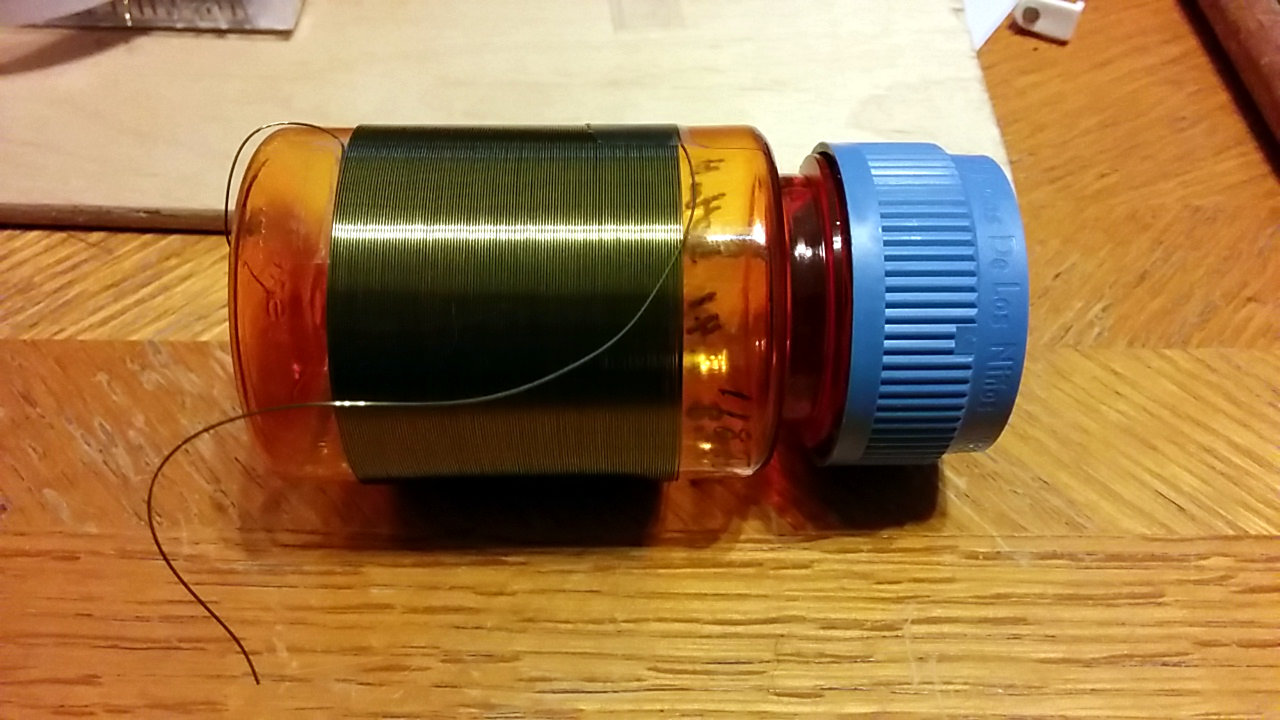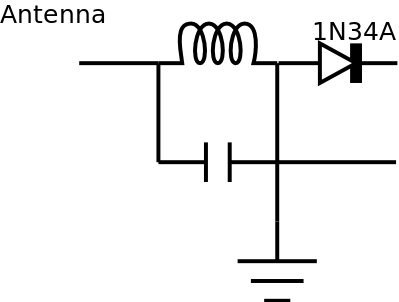# Coils

Time to see if I can build myself a crystal radio set. Or two. Or three.

I decided rather than demonstrate building a radio at the Charleston Library’s DIYFest, it would be easier to bring in some examples of some simple crystal radios that could be built using stuff easily obtained around the house. Found three nice cylindrical objects and started winding magnet wire.

The inductance of an air wound coil, according to Section 2.12.1 of the 2014 ARRL Handbook, is approximately

L (μH) = d2n2/(18d + 40l)

where d is the coil diameter in inches, l is the coil length in inches and n is the number of turns. Converting it to use more sensible metric units, it becomes

L (μH) = d2n2/(45.72d + 101.6l)

where d and l are in centimeters.

The first coil is 182 turns around a toilet paper tube. d = 4.5 cm, l = 6.7 cm, n = 182, and L = 757 μH.First coil – 182 turns, d=4.5cm l=6.7cm

Coil #2 is 110 turns around the cardboard core of a packing tape roll. d = 8 cm, l = 4 cm, n = 110, and L = 1003 μH.Coil #2 – 110 turns, d=8cm, l=4cm

Coil #3 is 118 turns around an empty plastic pill bottle. d = 4.8 cm, l = 4.1 cm, n = 118, and L = 504 μH.Coil #3, 118 turns, d=4.8cm, l=4.1cm

This is the basic schematic I’m planning on using.radio schematic

I want the radios to be able to tune in the AM broadcast band (540-1710 kHz) so the capacitance I’ll need for coils #1 and #2 is around 10 pF and around 17 pF for coil #3.

Since I have three coils, I think I’ll make one with a variable inductor, one with a variable capacitor, and one with a variable inductor and capacitor.

I don’t have any high impedance earpieces (I’ve seen some builds say you can use a telephone handset though), so I might add an amplifier or impedance matching transformer and see if I can get regular headphones to work.